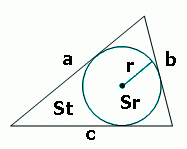homechevron_rightStudychevron_rightMathchevron_rightGeometry

# Incircle of a triangle

This online calculator determines the radius and area of the incircle of a triangle given the three sidesAnother triangle calculator, which determines radius of incircle

where, S, area of triangle, can be found using Hero's formula, p - half of perimeter.#### Incircle of a triangle

Digits after the decimal point: 2